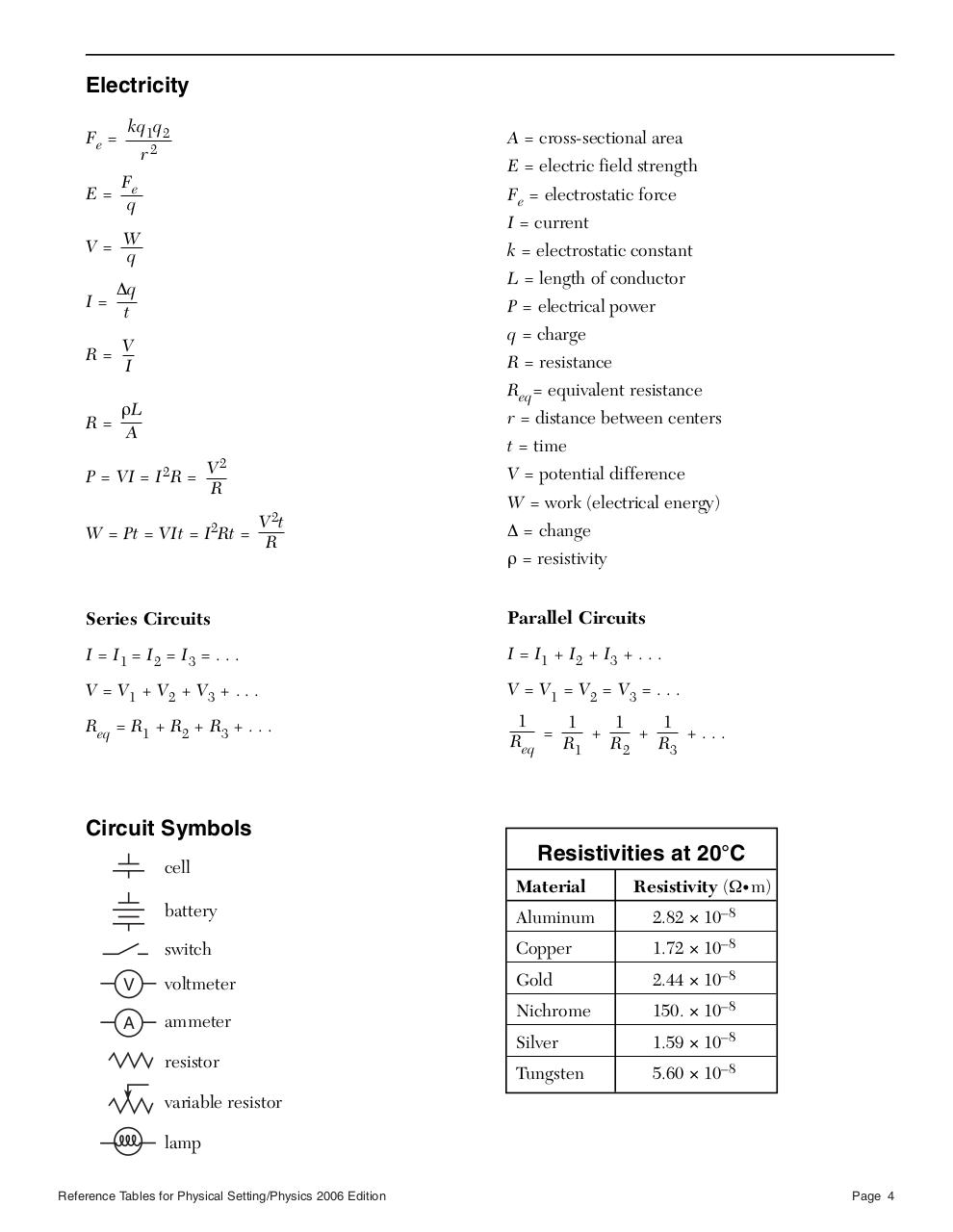# physics06tbl.pdfPage 1 2 3 4 5 6

#### Text preview

Electricity
Fe =

kq1q2

A = cross-sectional area

r2

E = electric field strength

F
E= e
q

Fe = electrostatic force
I = current

V= W
q

k = electrostatic constant
L = length of conductor

∆q
I=
t

P = electrical power
q = charge

R= V
I

R = resistance
Req= equivalent resistance

ρL
R=
A

r = distance between centers

P = VI = I 2R =

V2
R

V 2t
W = Pt = VIt = I2Rt =
R

t = time
V = potential difference
W = work (electrical energy)
∆ = change
ρ = resistivity

Series Circuits

Parallel Circuits

I = I1 = I2 = I3 = . . .

I = I1 + I2 + I3 + . . .

V = V1 + V2 + V3 + . . .

V = V1 = V2 = V3 = . . .

Req = R1 + R2 + R3 + . . .

1
1
1
1
Req = R1 + R 2 + R3 + . . .

Circuit Symbols
cell

Resistivities at 20°C
Material

Resistivity (Ω• m)

battery

Aluminum

2.82 × 10–8

switch

Copper

1.72 × 10–8

V

voltmeter

Gold

2.44 × 10–8

A

ammeter

Nichrome

150. × 10–8

Silver

1.59 × 10–8

Tungsten

5.60 × 10–8

resistor
variable resistor
lamp
Reference Tables for Physical Setting/Physics 2006 Edition

Page 4